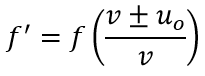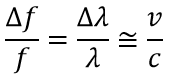# 9.1 Simple Harmonic Motion (SHM)

• Period of the motion is related to the angular frequency (ω), not to the amplitude or to the phase. T = 2π/ω.

• Acceleration is proportional and in the opposite direction to the displacement. a = -ω²x.

Spring

• Restoring force: After being moved a distance A (Amplitude) from equilibrium point, there will be a restoring force towards the center.

• When there are two springs, the force doubles!​

• Period is independent of the amplitude/extension.​

Simple pendulum

• Small angle approximation: Acceleration is not proportional to the displacement (x). But if x is small (x < 10º) then sin(x/L) is approximately equal to x/L.

• Period is independent of the mass.

Formulas

When at t = 0, the displacement equals the amplitude:

• x = xo cos(ωt);

• v = -ωxo sin(ωt);

• a = -ω²xo cos(ωt) = -ω²x.

When at t = 0, the displacement (x) equals zero:

• x = xo sin(ωt);

• v = -ωxo cos(ωt);

• a = -ω²xo sin(ωt) = -ω²x.

Maximums: The maximum speed is ωxo and maximum acceleration is ω²xo.

Circular motion and SHM

Energy in SHM systems

• Total energy conservation: ET = EK + EP.

• ET = 1/2 mω²xo².

• EK = 1/2 mv² = 1/2 mω² xo² sin²(ωt) = 1/2 mω² (xo² - x²). ​

• EP = 1/2 mω² xo² cos²(ωt) = 1/2 mω²x².

# 9.2 Single-slit Diffraction

#### Diffraction is appreciable if the wavelength is of the same order of magnitude as the opening (b) or bigger, i.e. λ ≥ b.​

• Central maximum is twice the width of other maxima, which are evenly separated.

• Central maximum is about 20 times as intense as the other maxima.

Variation of intensity with angle for a diffraction pattern

• Central maximum is twice the width of other maxima, which are evenly separated.

• Central maximum is about 20 times as intense as the other maxima.

• Each successive maxima (excluding the central maximum) has less than half the previous one's intensity.

Diffraction by a single rectangular slit

• Huygen's principle: You may always think on a wavefront as an infinite series of source points, generating secondary waves, which, summed together, equal the total wavefront.

• Single-slit diffraction explanation: "For two waves coming from the edges of the slit making an angle θ with the straight through, there is a path difference bsinθ. Waves from a point halfway along the slit will have a path difference of b/2 sinθ". (Bowen-Jones & Homer, 2014)

• Consequence: For each point in the bottom half of the slit, there will be a point in the upper half of the slit with a path difference of b/2 sinθ.

• Small angle approximation: ​tanθ = sinθ = θ, and thus, b/2 sinθ = bθ/2 =  λ/2, i.e. bθ = λ.

• θ = s/D, where D is the distance between the slit and screen and s = distance of first minimum from center.

• Destructive interference: ​When the path difference equals half the wavelength.

• First minimum: θ = λ/b.

• Additional minima: θ = nλ/b, n = 1, 2, 3...

• Diffraction with laser is monochromatic.

• Diffraction with white light: central maximum is white, fringes will be colored and blue diffracts the least.

# 9.3 Interference

#### Double-slit interference

• Sources must be exactly in phase, otherwise no interference pattern will be formed.

• Destructive interference: If the path difference is a half-integral multiple of λ.

• Path difference = (n +1/2)λ, n = 0, 1, 2...

• Constructive interference: If the path difference is an integral multiple of λ.

• Path difference = dsinθ = nλ, n = 0, 1, 2..., where θ is the angle between two primary maxima.

#### Intensity variation:

• Slit widths of negligible size:

• Bright fringes are equally bright and spaced.

• Smaller slit separation: maxima are further apart.

• Slit widths with real size: modulation by single-slit diffraction.

• Constant fringe spaging, varying intensity.

#### Multiple slits (N)

• With N slits there are N-2 secondary maxima between primary maxima.

• Intensity of the central maximum is N².

• Primary maxima of the multiple-slit interference pattern are observed at the same angles as the corresponding two-slit pattern with the same slit separation.

• As N increases:

• Primary maxima become thinner and sharper.

• Secondary maxima become unimportant.

Three slits:

#### Diffraction grating: large number of parallel slits, whose width we take to be negligible.

• Uses: analyze wavelength of light, disperse white light into its component colors.

• Maxima are sharp and bright, as there is a large number of slits causing constructive interference.

• Spacing between the slits is small, and thus, there is no small angle approximation, as the angular separation of the maxima is very large. dsinθ = nλ, where n is known as the "order" and n = 0, 1, 2..., i.e. 0 order, 1st order, 2nd order...

• Maximum order: Found using sin​θ < 1, when d and λ are known.

• Total number of orders: 2n + 1 (Counting "0 order" and the negative orders).

• Lines per millimeter: diffraction grating is stated to have N lines per millimeter. This means that the separation of the slits is d = 1/N mm.

#### Interference may occur by:

• Division of wavefronts: taking waves from different parts of the same wavefront, and thus, they are in phase. Called "non-localized", as they may be found anywhere.

• Division of amplitude: achieving interference using two waves that come from the same point on a wavefront. The source of waves must be much bigger than the slit used for division. Image produced by the two waves, each with a portion of the original one, is localized to one plane.

#### Thin film interference:

• Example: reason why thin films, such as soap bubbles, show colorful patterns.

• Explanation: upon reflection off a thin film, a ray of light will undergo a phase change of π, if it reflects off a medium of higher refractive index (arrives as a crest, reflects as a trough).

• Perpendicular: if the angle with the normal is small, as one was looking at a thin film perpendicularly, the path difference will be 2d.

• Constructive interference: 2dn = (m + 1/2)λ, where n is the refractive index.

• Phase change of π included in the formula (+1/2).

• Destructive interference: 2dn = mλ.

• White light: the wavelength that suffers destructive interference will have the corresponding color absent in the reflected light, giving the pattern seen in soap bubbles.

# 9.4 Resolution

#### Resolution

• Explanation: the ability of an imaging system (e.g. eyes, camera or telescope) to be able to produce two separate distinguishable images of two separate objects.

• Diffraction: light from each of the objects will diffract as it goes through the opening of the imaging system. This creates diffraction patterns. ​

• Rayleigh criterion (minimum for images - diffraction patterns - to be just resolved):​ "Two sources are just resolved if the principal (central) maximum from one diffraction pattern is no closer than the first minimum of the other pattern".

• Angular separation (θA) = s/d, where s is the distance between the central maxima of the diffraction patterns of the objects and d the distance between the observer and the objects.

• According to Rayleigh criterion, resolution is only possible when: ​θA ≥ θD.

• For a circular slit: θ= 1.22λ/b, where b is the diameter of the slit and θD is known as the diffraction angle.

• For a rectangular slit: θ= λ/b.

#### Diffraction grating resolvance (R)

• Definition: the ratio of the wavelength of the light to the smallest difference in wavelength that can be resolved by the grating λ. R = λ/λ.

• Explanation: ability to see as distinct two lines in a spectrum that correspond to wavelengths λ1 and λ2 that are very close to each other. Hence, R = λaverage/λ, where λaverage = (λ1 + λ2)/2

• R = Nm, where N is the total number of slits illuminated by the incident beam and m is the order of diffraction.

• Number of lines: When the incident beam is x millimeter wide, the number of lines is N/x.

# 9.5 The Doppler Effect

#### Definition: "Doppler effect is the change in the observed frequency of a wave when there is relative movement when there is relative movement between the source and the observer."

Wavefront diagrams

#### Cases

Quantities:

• f = Frequency of the source;

• f' =Apparent frequency, heard by the observed;

• v = Speed of the wave;

• us Velocity of the source;

• uVelocity of the observer.

Moving source and stationary observer:• - us for a source moving towards a stationary observer. f' > f, because λ are shorter, as the wavefronts are being compressed.

• + us for a source moving away from a stationary observer. f' < f, because λ are longer.

• v, as measured by the observer, is v, since it is determined by the medium's properties.

Stationary source and moving observer:• λ remains equal and v changes, as the medium changed: source - air; observer - moving air.

• - uo for an observer moving away from a stationary source. f' < f, but measured λ is constant.

• + uo for an observer moving towards a stationary source. f' > f, but measured λ is constant.

#### Examples

• Police speed radar: car moving away, reflecting microwaves from the source.

• Medical physics: blood flow measurement

The pumping of the heart makes the blood have speed "waves", which are detectable as a sort of beat frequency.

The machine detects when that expected speed (from the beat frequency) varies, due to constriction or obstruction of the vessel.

#### Doppler effect with light​• Formula different for sound, as the velocity of light is constant in all inertial reference frames (by special relativity), which means that, when measured by an observed who is not accelerating, the observer will measure the speed of light to be 3 x 10^8 ms^-1.

• Blue-shift: If the source of light approaches, then the frequency increases and the wavelength decreases.

• Red-shift: If the source of light moves away, then the frequency decreases and the wavelength increases.

• Expanding Universe proof: Light from distant galaxies measured on Earth shows a red-shift, what indicates galaxies are moving away from us, and thus, the universe is expanding.

Red-shift